Last Updated: June 2020

Purpose: To provide users with some of the commonly used equations for the Wave Sensor as well as provide other equations that may be helpful in implementing the sensor for the specific end-user application.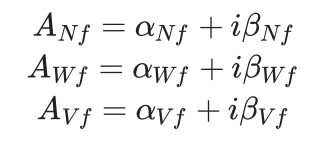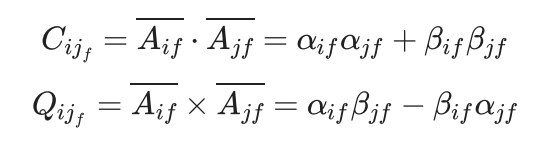Fourier coefficient may be calculated for each frequency bin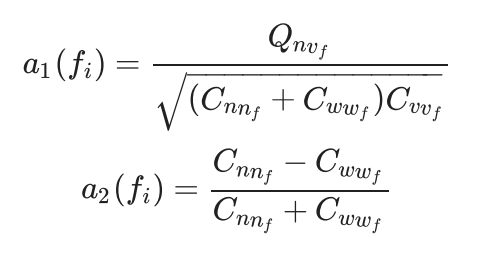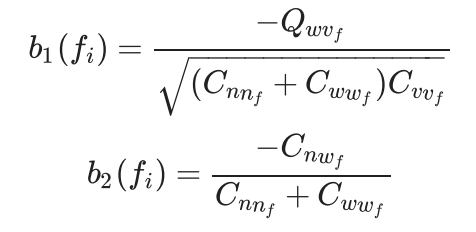Wave Mean Direction- The mean direction from which the waves are coming or going as a function of frequency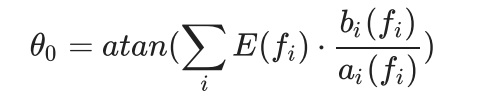Mean Wave Direction Spectrum- The mean distribution of wave energy in wave number and direction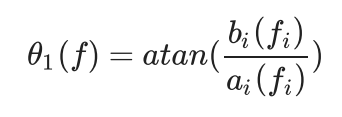Principal Wave Direction Spectrum- Equation of principal wave direction is similar to mean wave direction, but it is calculated from other directional Fourier series coefficients.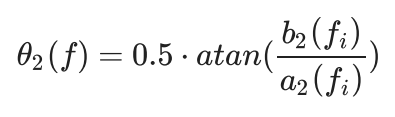Significant Frequency- the frequency at which the harmonic components of the real waveform begin to drop off faster than 1/f.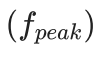Wave Fourier Coefficients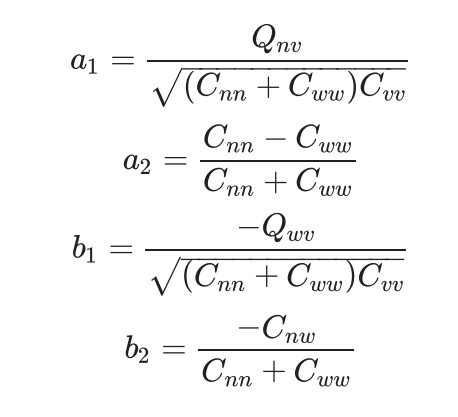Wave Peak Direction- The wave direction at the frequency at which a wave energy spectrum reaches its maximum.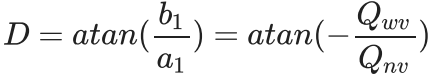Wave Peak Period- The wave period associated with the most energetic waves in the total wave spectrum at a specific point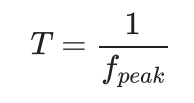Mean Spreading Angle- Angle at which even spreading is assumed across the entire frequency range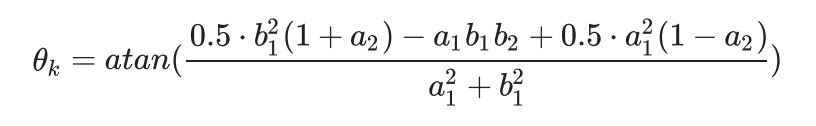Directional Width- indicates whether waves are coming from similar directions or a wide range of directions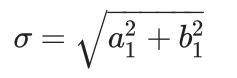Long Crestedness Parameter- measures the surface texture of the wave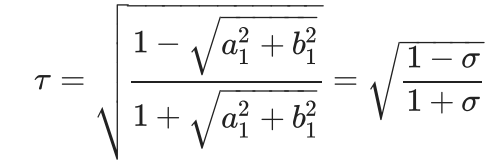Topics: Sensor, Wave, Equations

• Content Title

Description